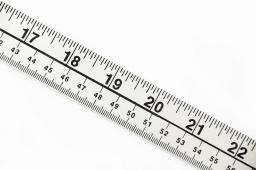# Equation: 5482

Solve the equation:

0.5 • (3+ x) -0.2 • (0.5x-5) = 0Did you find an error or inaccuracy? Feel free to write us. Thank you!

Tips for related online calculators
Do you have a linear equation or system of equations and looking for its solution? Or do you have a quadratic equation?